# DAV Class 6 Maths Chapter 14 Worksheet 1 Solutions

The DAV Class 6 Maths Book Solutions and DAV Class 6 Maths Chapter 14 Worksheet 1 Solutions of Constructions offer comprehensive answers to textbook questions.

## DAV Class 6 Maths Ch 14 WS 1 Solutions

Question 1.
Draw a line segment XY equal to 8 cm. Using compass and ruler, construct its perpendicular bisector.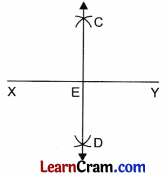Step 1: Draw XY = 8 cm.
Step 2: Take X and Y as centres and draw two arc with a radius greater than $$\frac{1}{2}$$ of XY above and below the segment XY.
Step 3: The two arcs intersect each other at C and D.
Step 4: Join CD and produce in both directions which meets XY at E. CD is the required perpendicular bisector of XY at E.

Question 2.
Draw a line segment PQ equal to 7 cm. Using ruler and compass, obtain a line segment of length i PQ. Measure each part.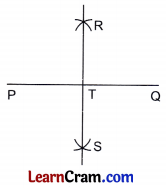Step 1: Draw PQ = 7 cm.
Step 2: Take P and Q as centres and draw two arcs with radius more than $$\frac{1}{2}$$ × PQ to intersect each other at R and S above and below the segment PQuestion
Step 3: Join R and S which intersects PQ at T.
Step 4: Measure PT and TQ, which is equal to 3.5 cm.
Hence, measure of each equal part is 3.5 = $$\frac{1}{2}$$ × PQQuestion 3.
Draw a circle of radius 6 cm. Draw its diameter and name it AB. Using compasses and ruler, construct the perpendicular bisector of AB. Does it pass through the centre of the circle?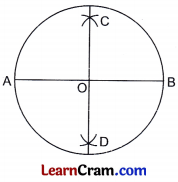Step 1 : Draw a circle with centre O and radius 6 cm.
Step 2: Draw any diameter AB.
Step 3: Taking A and B as centres, draw two arcs with the radius more than half of AB above and below the segment AB.
Step 4: The two arcs meet each other at C and D.
Step 5: Join CD and we observe that CD passes through the centre O of the circle.

### DAV Class 6 Maths Chapter 14 Worksheet 1 Notes

1. Construction of Angles:
For the construction of angles we generally use scale, pencil and protractor.
We use ruler, compass and pencil to construct the following angles:
15°, 30°, 45°, 105°, 120°, 135°, 22$$\frac{1}{2}$$°, 67$$\frac{1}{2}$$° etc.

2. Construction of the perpendicular bisector of a given line segment:Step 1: Draw a line segment PQ.
Step 2: Take P as a centre and draw an arc equal to the radius more than half of PQ above and below the segment PQ.
Step 3: Draw another arc with centre Q and of same radius.
Step 4: Join the point of intersection of the two arcs which is the required perpendicular bisector of PQ.

3. Construction of an angle equal to the given angle: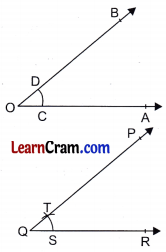Step 1: Draw an arc CD in the given angle AOB with O as centre.
Step 2: Draw a ray QR and draw an arc with centre Q and radius equal to OC which meets QR at S.
Step 3: With centre S cut an arc with a radius equal to CD which cut the first arc at T.
Step 4: Join QT and produce to P.
Step 5: ∠PQR is the required angle equal to ∠AOB.

4. Construction of the bisector of a given angle: ∠AOB is the given angle.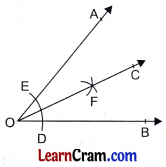Step 1 : Take O as centre and draw an arc of suitable radius which meets the two arms of the angle at D and E.
Step 2: Draw two arcs with centres D and E with a radius more than half of arc DE.
Step 3: The two arcs intersect each other at F.
Step 4: Join OF and produce to C.
Step 5: OC is the required bisector of ∠AOB such that ∠AOC = ∠COB